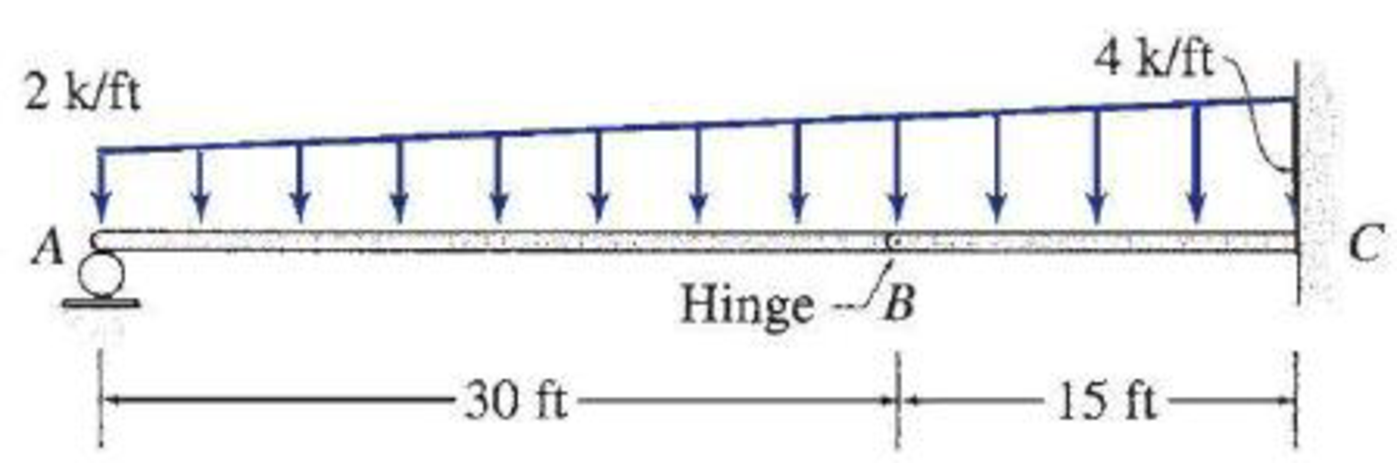# 5.12 through 5.28 Determine the equations for shear and bending moment for the beam shown. Use the resulting equations to draw the shear and bending moment diagrams.

#### Solutions

Chapter
Section
Chapter 5, Problem 26P
Textbook Problem
34 views

## 5.12 through 5.28 Determine the equations for shear and bending moment for the beam shown. Use the resulting equations to draw the shear and bending moment diagrams.To determine

Find the equations for the shear and bending moment of the beam.

Plot the shear and bending moment diagram.

### Explanation of Solution

Sign conversion:

Apply the sign convention for calculating the equations of equilibrium as below.

• For the horizontal forces equilibrium condition, take the force acting towards right side as positive (+) and the force acting towards left side as negative ().
• For the vertical forces equilibrium condition, take the upward force as positive (+) and downward force as negative ().
• For moment equilibrium condition, take the clockwise moment as negative and counter clockwise moment as positive.

Apply the following sign convention for calculating the shear and bending moments.

• When the portion of the beam considered is left of the section, then the external force acting to the left are considered as positive.
• When the portion of the beam considered is right of the section, then the external force acting to the right are considered as positive.
• When the portion of the beam considered is left of the section, then the external force acting upward are considered as positive.
• When the portion of the beam considered is right of the section, then the external force acting downward are considered as positive.
• When the portion of the beam considered is left of the section, then the clockwise moments are considered as positive.
• When the portion of the beam considered is right of the section, then the counterclockwise moments are considered as positive.

Calculation:

Show the free-body diagram of the entire beam as in Figure 1.

Consider the section AB and take moment about the hinge at B.

Find the vertical reaction at point A by taking moment about point B.

+MBAB=0Ay(30)+2(30)(302)+12×(1032)×30×303=030Ay+900+200=0Ay=36.67k

Find the horizontal reaction at point C by resolving the horizontal equilibrium.

+Fx=0Cx=0

Find the moment at point C by taking moment about point C.

+MC=0Ay(45)+2(45)(452)+12×(42)×45×453MC=036.67(45)+2025+675MC=0MC=1050k-ft

Find the vertical reaction at point C by resolving the vertical equilibrium.

+Fy=0Ay2×4512×(42)×45+Cy=036.679045+Cy=0Cy=98.33k

Pass the section aa at a distance x from left end of the beam.

Show the section aa in the entire beam as in Figure 2

### Still sussing out bartleby?

Check out a sample textbook solution.

See a sample solution

#### The Solution to Your Study Problems

Bartleby provides explanations to thousands of textbook problems written by our experts, many with advanced degrees!

Get Started

Find more solutions based on key concepts
Your future company has purchased a machine and has entered into a contract that requires the company to pay 20...

Engineering Fundamentals: An Introduction to Engineering (MindTap Course List)

What is an advantage of using locking pliers?

Precision Machining Technology (MindTap Course List)

Principles of Information Security (MindTap Course List)

tethering a. text-based name that corresponds to the IP address of a server that hosts a website b. website or ...

Enhanced Discovering Computers 2017 (Shelly Cashman Series) (MindTap Course List)

Define dirty data, and identify some of its sources.

Database Systems: Design, Implementation, & Management

If your motherboard supports ECC DDR3 memory, can you substitute non-ECC DDR3 memory?

A+ Guide to Hardware (Standalone Book) (MindTap Course List)